JEE  >  Ionic Equilibrium MCQ - 1 (Advance)

# Ionic Equilibrium MCQ - 1 (Advance)

Test Description

## 20 Questions MCQ Test Chemistry for JEE Advanced | Ionic Equilibrium MCQ - 1 (Advance)

Ionic Equilibrium MCQ - 1 (Advance) for JEE 2022 is part of Chemistry for JEE Advanced preparation. The Ionic Equilibrium MCQ - 1 (Advance) questions and answers have been prepared according to the JEE exam syllabus.The Ionic Equilibrium MCQ - 1 (Advance) MCQs are made for JEE 2022 Exam. Find important definitions, questions, notes, meanings, examples, exercises, MCQs and online tests for Ionic Equilibrium MCQ - 1 (Advance) below.
Solutions of Ionic Equilibrium MCQ - 1 (Advance) questions in English are available as part of our Chemistry for JEE Advanced for JEE & Ionic Equilibrium MCQ - 1 (Advance) solutions in Hindi for Chemistry for JEE Advanced course. Download more important topics, notes, lectures and mock test series for JEE Exam by signing up for free. Attempt Ionic Equilibrium MCQ - 1 (Advance) | 20 questions in 40 minutes | Mock test for JEE preparation | Free important questions MCQ to study Chemistry for JEE Advanced for JEE Exam | Download free PDF with solutions
 1 Crore+ students have signed up on EduRev. Have you?
*Multiple options can be correct
Ionic Equilibrium MCQ - 1 (Advance) - Question 1

### Which of the following statement(s) is/are correct?

Detailed Solution for Ionic Equilibrium MCQ - 1 (Advance) - Question 1

(A)
pH of 10–8 m sol. of HCl is 6.97 (consider the H+ from H2O also) (B)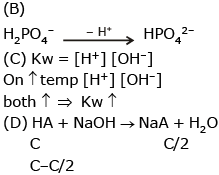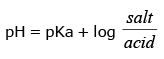*Multiple options can be correct
Ionic Equilibrium MCQ - 1 (Advance) - Question 2

### A 2.5 gm impure sample containing weak monoacidic base (Mol. wt. = 45) is dissolved in 100 ml water and titrated with 0.5 M HCl when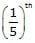of the base was neutralised the pH was found to be 9 and at equivalent point pH of solution is 4.5. Given : All data at 25º C & log 2 = 0.3. Select correct statement(s).

Detailed Solution for Ionic Equilibrium MCQ - 1 (Advance) - Question 2

BOH + HCl  → BCl + H2O
100 m 0.5 V 20 M
100 M – 0.5 V  0  = 80 M
14–a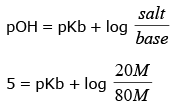⇒ pkb = 5 – log 0.25 ⇒ pkb = 5.6
⇒ Kb = 2.5 × 10–6
greater than 10–6

*Multiple options can be correct
Ionic Equilibrium MCQ - 1 (Advance) - Question 3

### Select incorrect statement(s).

Detailed Solution for Ionic Equilibrium MCQ - 1 (Advance) - Question 3

At eq. point pH = 4.5
pOH = 9.5
pOH = 1/2 [Pkb + pkw + hc ]
9.5 = 1/2 [5.6 + 14 + hc] ⇒ C = 0.25
(C) Total volume be (100 + V)
0.25 × (100 + V) = 0.5 × C  ⇒  V = 100 ml (D) 100 M = 0.5 V
100 × M = 0.5 × 100
M = 0.5
100 ml _________ 0.5 mole
100 ml _________ 0.055 mole of base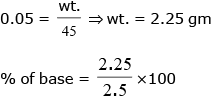90%

*Multiple options can be correct
Ionic Equilibrium MCQ - 1 (Advance) - Question 4

Which of the following is true for alkaline aqueous solution?

Detailed Solution for Ionic Equilibrium MCQ - 1 (Advance) - Question 4

For alkaline solution
pH > 7

Ionic Equilibrium MCQ - 1 (Advance) - Question 5

Ionisation constant of formic acid is 1.8 * 1.8*10−4 at 298 K. In 0.1 NHCOOH the percentage ionisation of HCOOH acid is

Ionic Equilibrium MCQ - 1 (Advance) - Question 6

A solution contains HCl, Cl2HC COOH & CH3COOH at concentation 0.09 M in HCl, 0.09 M in Cl2HC COOH & 0.1 M in CH3COOH. pH for the solution is 1. Ionization constant of CH3COOH = 105. What is the magnitude of K for dichloroacetic acid ?

Detailed Solution for Ionic Equilibrium MCQ - 1 (Advance) - Question 6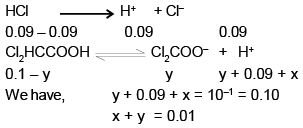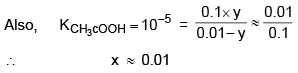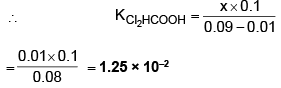Ionic Equilibrium MCQ - 1 (Advance) - Question 7

A solution of chloroacetic acid, ClCH2COOH containing 9.45 grams in 500 ml of the solution has a pH of 2.0. What is the degree of ionization the acid.

Detailed Solution for Ionic Equilibrium MCQ - 1 (Advance) - Question 7

MW of ClCH2COOH = 44.5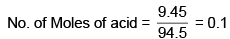∴ [acid] = 0.1 × 2 = 0.2 M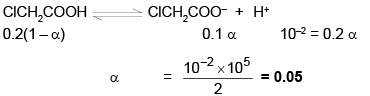Ionic Equilibrium MCQ - 1 (Advance) - Question 8

The Kw of water at two different temperature is :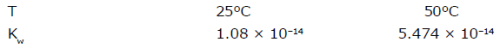Assuming that ΔH of any reaction is independent of temperature, calculate the enthalpy of neutralization of strong acid and strong base.

Detailed Solution for Ionic Equilibrium MCQ - 1 (Advance) - Question 8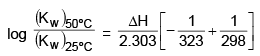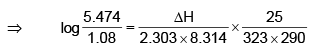(ΔH)ionization of H2O = 51.963 KJ/mol
∴ (ΔH)Neutralization  = – 51.963 KJ/mol

Ionic Equilibrium MCQ - 1 (Advance) - Question 9

The equilibrium constant of the reaction.

2Ag(s) + 2I- + 2H22Agl(s) + H2(g) + 2OH-

is 1.2 × 10-23 at 25°C. Calculate the pH of a solution at equilibrium with the iodine ion concentation = 0.10 and the pressure of H2 gas = 0.60 atm.

Detailed Solution for Ionic Equilibrium MCQ - 1 (Advance) - Question 9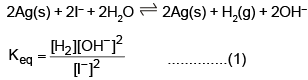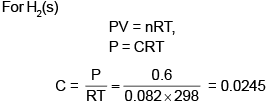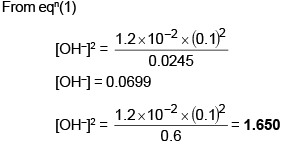Ionic Equilibrium MCQ - 1 (Advance) - Question 10

When a 40 mL of a 0.1 M weak base in titrated with 0.16 M HCl, the pH of the solution at the end point is 5.23. What will be the pH if 15 mL of 0.12 M NaOH is added to the resulting solution.

Detailed Solution for Ionic Equilibrium MCQ - 1 (Advance) - Question 10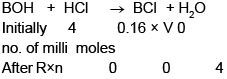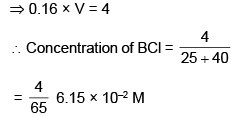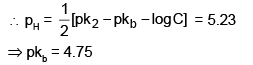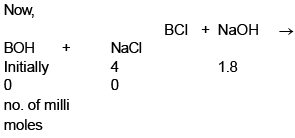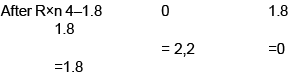The mixture is buffer of BOH and BCl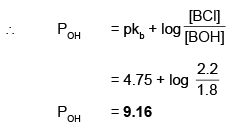Ionic Equilibrium MCQ - 1 (Advance) - Question 11

How many moles of sodium hydroxide can be added to 1.00 L of a solution 0.1 M in NH3 & 0.1 M in NH4Cl without changing the pOH by mor than 1.00 unit ? Assume no change in volume. Kb(NH3) = 1.8 × 10-5.

Detailed Solution for Ionic Equilibrium MCQ - 1 (Advance) - Question 11

NH4Cl →  NH4+ + Cl
NH3 + H2O ® NH4+ + OH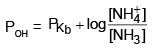= 5 –log 1.8 + log(1)
= 4.74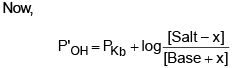where x is moles of NaOH added
⇒ P'OH -PKb = P'OH -POH = 1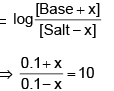⇒ 0.1 + x = 1 – 10x
⇒ 11 x = 0.9
⇒ x  = 0.0818 moles

Ionic Equilibrium MCQ - 1 (Advance) - Question 12

20 ml of a solution of 0.1 M CH3COOH solution is being titrated against 0.1 M NaOH solution. The pH vaues after the addition of 1 ml & 19 ml of NaOH are (pH)1 & (pH)2, what is ΔpH ?

Detailed Solution for Ionic Equilibrium MCQ - 1 (Advance) - Question 12

CH3COOH + NaOH → CH3COONa + H2O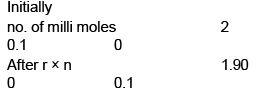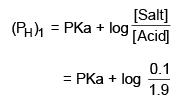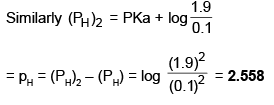Ionic Equilibrium MCQ - 1 (Advance) - Question 13

Calculate the OH- concentration and the H3PO4 concentration of a solution prepared by dissolving 0.1 mol f Na3PO4 in sufficient water to make 1 l of solution. K1 = 7.1 × 10-3, K2 = 6.3 × 10-8, K3 = 4.5 × 10-13.

Detailed Solution for Ionic Equilibrium MCQ - 1 (Advance) - Question 13

Na3PO4 → 3Na+ + PO43–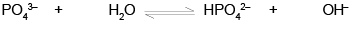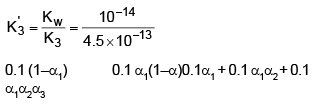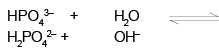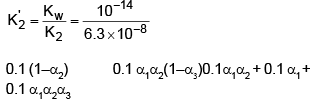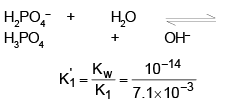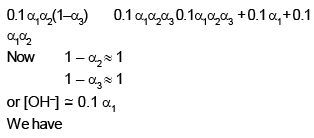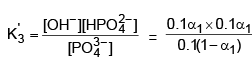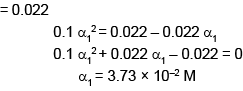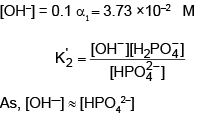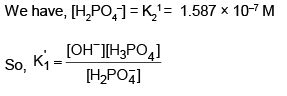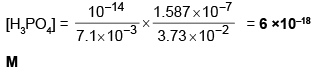Ionic Equilibrium MCQ - 1 (Advance) - Question 14

Solubility product of AgCl is 2.8 × 10-10 at 25ºC. Calculate solubility of the salt in 0.1 M AgNO3 solution -

Detailed Solution for Ionic Equilibrium MCQ - 1 (Advance) - Question 14

Ksp(AgCl) = 2.8 × 10–10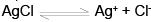2.8 × 10–10 = (s + 0.1)s
s = 2.8 × 10–9

Ionic Equilibrium MCQ - 1 (Advance) - Question 15

Equilibrium constant for the acid ionization of Fe3+ to Fe(OH)+2 and H+ is 6.5 × 10-3. What is the max. pH, which could be used so that at least 95% of the total Fe3+ in a dilute solution. exists are Fe3+.

Detailed Solution for Ionic Equilibrium MCQ - 1 (Advance) - Question 15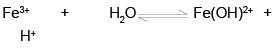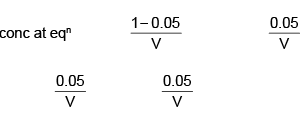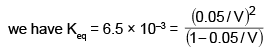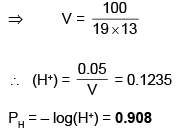Ionic Equilibrium MCQ - 1 (Advance) - Question 16

A solution of weak acid HA was titrated with base NaOH. The equivalence point was reaced when 36.12 ml of 0.1 M NaOH has been added. Now 18.06 ml of 0.1 M HCl were added to titration solution, the pH was found to be 4.92. What will be the pH of the solution obtained by mixing 10 ml 0.2 M NaOH and 10 ml of 0.2 M HA.

Detailed Solution for Ionic Equilibrium MCQ - 1 (Advance) - Question 16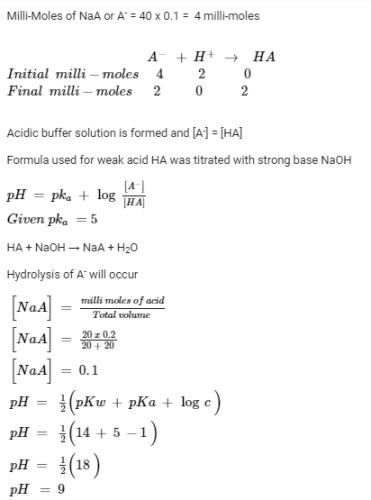Ionic Equilibrium MCQ - 1 (Advance) - Question 17

A weak base BOH was titrated against a strong acid. The pH at 1/4 the equivalence point was 9.24. Enough strong base was now added (6m eq.) to completely convert the salt. The total volume was 50 ml. Find the pH at this point.

Detailed Solution for Ionic Equilibrium MCQ - 1 (Advance) - Question 17

BOH + HCl → BCl + H2O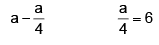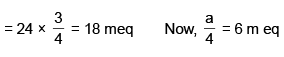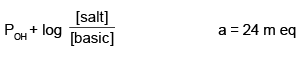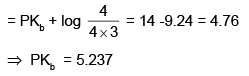Now, BCl + NaOH → BOH + NaCl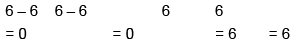Total BOH = 6 + 18 = 24 m eq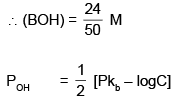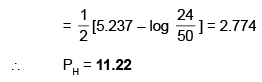Ionic Equilibrium MCQ - 1 (Advance) - Question 18

At 25°C, will a precipitate of Mg(OH)2 form in a 10-4 M solution of Mg(NO3)2 if pH of the solution is adjusted to 9.0. Ksp [Mg(OH)2] = 10-11 M3. At what min value of pH will precipitation start.

Detailed Solution for Ionic Equilibrium MCQ - 1 (Advance) - Question 18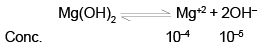As, Ionic product, 10–4 × (10–5)2 < Ksp
No ppt occurs.
Min  value of [OH–] for pptn to occur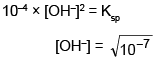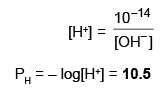Ionic Equilibrium MCQ - 1 (Advance) - Question 19

What is the solubility of AgCl in 0.20 M NH3 ?

Given : Ksp(AgCl) = 1.7 × 10-10 M2, K1 = [Ag(NH3)+] / [Ag+] [NH3] = 2.33 × 103M-1 and

K2 = [Ag(NH3)2+] / [Ag (NH3)[NH3] = 7.14 × 103 M-1

Detailed Solution for Ionic Equilibrium MCQ - 1 (Advance) - Question 19

If x be the concentration of AgCl in the solution, then [Cl] = x
From the Ksp for AgCl, we derive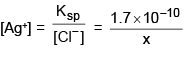If we answer that the majority of the dissolved
Ag+ goes into solution as Ag(NH3)2+ then
Ag(NH3)2+ then
Ag(NH3)2+ = x
Since two molecules of NH3 are required for every Ag(NH3)2+ ion formed, we have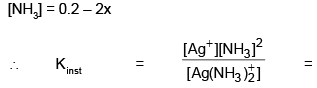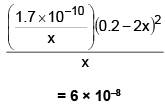∴  x = [Ag(NH3)2+] = 9.6 × 10–3 M, which is the solubility of AgCl in 0.2 M NH3

Ionic Equilibrium MCQ - 1 (Advance) - Question 20

Equal volumes of 0.02 M AgNO3 and 0.02 M HCN were mixed. Calculate [Ag+] at equilibrium. Take Ka(HCN) = 9 × 10-10, Ksp (AgCN) =4 × 10-6.

Detailed Solution for Ionic Equilibrium MCQ - 1 (Advance) - Question 20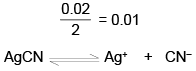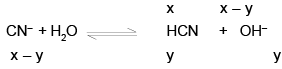W e have, Ksp(AgCN) = x (x – y) = 4 × 10–16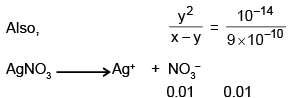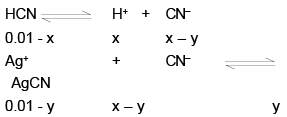(0.01-y) (x-y) = Ksp(AgCN) = 4 × 106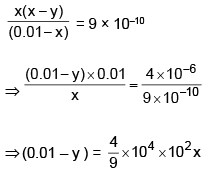## Chemistry for JEE Advanced

12 videos|53 docs|118 tests
 Use Code STAYHOME200 and get INR 200 additional OFF Use Coupon Code
Information about Ionic Equilibrium MCQ - 1 (Advance) Page
In this test you can find the Exam questions for Ionic Equilibrium MCQ - 1 (Advance) solved & explained in the simplest way possible. Besides giving Questions and answers for Ionic Equilibrium MCQ - 1 (Advance), EduRev gives you an ample number of Online tests for practice

## Chemistry for JEE Advanced

12 videos|53 docs|118 tests